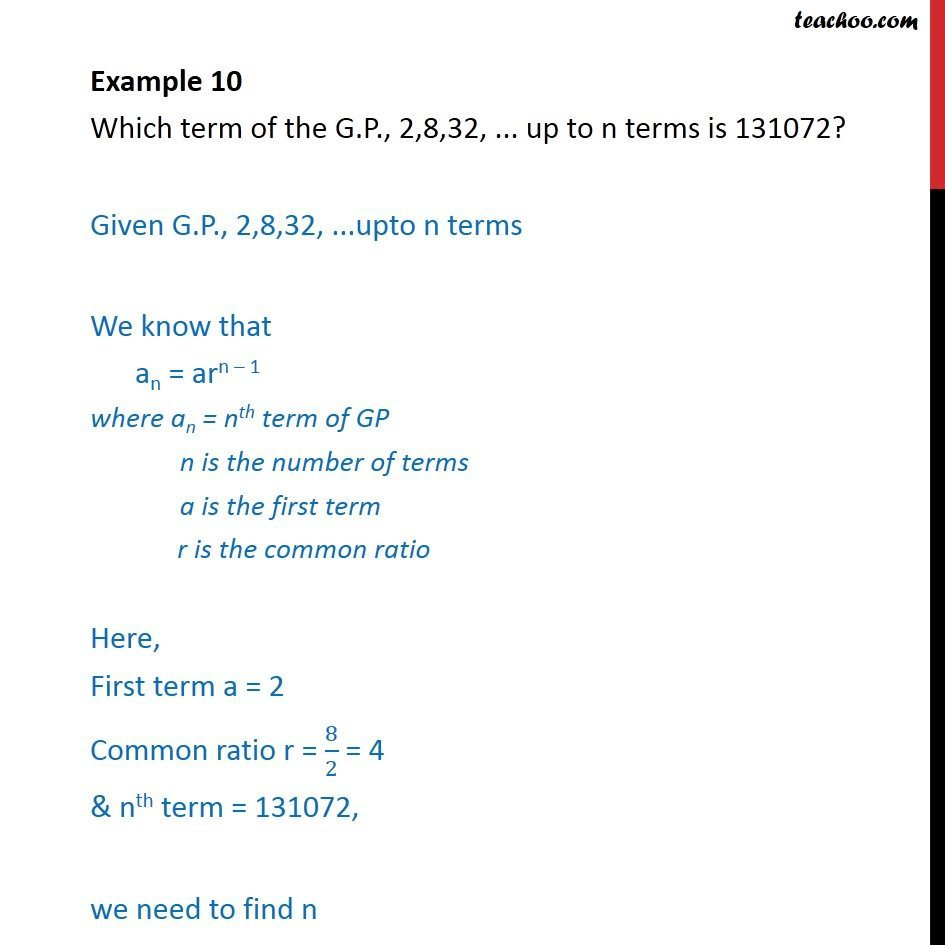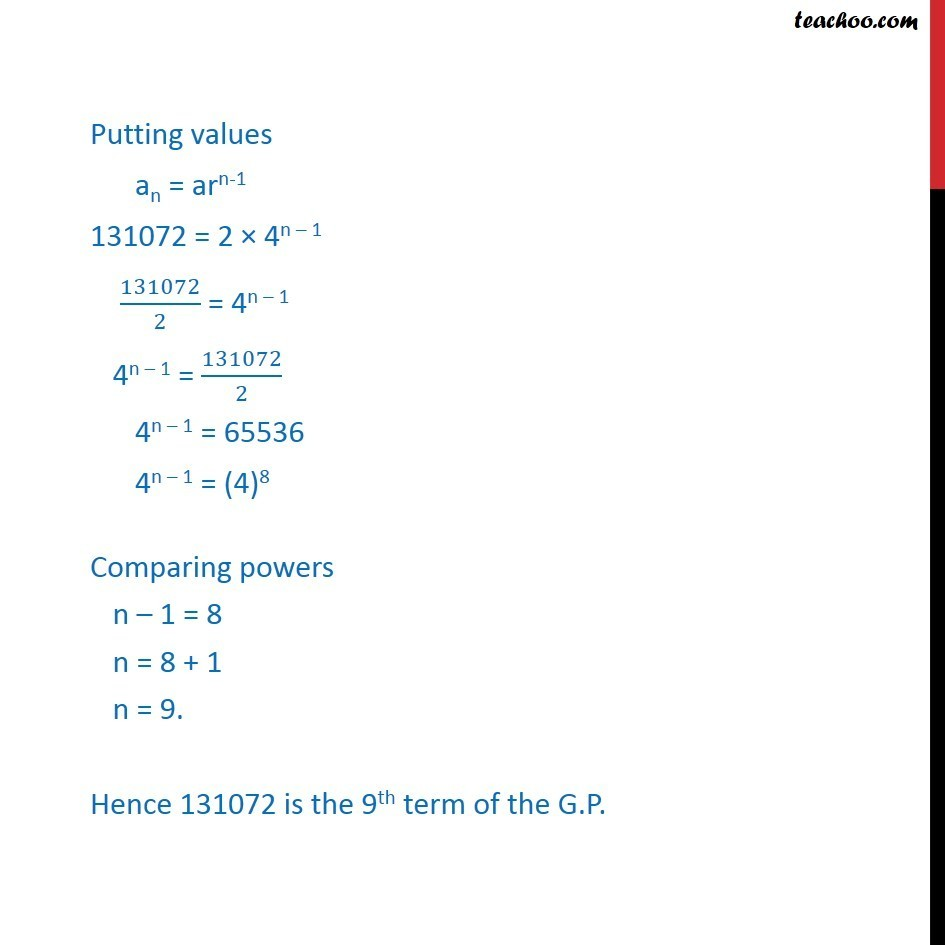Geometric Progression(GP): Formulae based

Chapter 8 Class 11 Sequences and Series
Concept wiseLearn in your speed, with individual attention - Teachoo Maths 1-on-1 Class

### Transcript

Example 5 Which term of the G.P., 2,8,32, ... up to n terms is 131072? Given G.P., 2,8,32, ...upto n terms We know that an = arn – 1 where an = nth term of GP n is the number of terms a is the first term r is the common ratio Here, First term a = 2 Common ratio r = 8/2 = 4 & nth term = 131072, we need to find n Now an = 131072 arn-1 = 131072 2 × 4n – 1 = 131072 4n – 1 = 131072/2 Putting values an = arn-1 131072 = 2 × 4n – 1 131072/2 = 4n – 1 4n – 1 = 131072/2 4n – 1 = 65536 4n – 1 = (4)8 Comparing powers n – 1 = 8 n = 8 + 1 n = 9. Hence 131072 is the 9th term of the G.P.